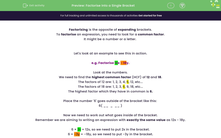# Factorise Into a Single Bracket

In this worksheet, students will factorise expressions (i.e. add brackets in) by finding highest common factors in terms of numbers.Key stage:  KS 4

Year:  GCSE

GCSE Subjects:   Maths

GCSE Boards:   Pearson Edexcel, OCR, Eduqas, AQA,

Curriculum topic:   Algebra

Curriculum subtopic:   Notation, Vocabulary and Manipulation Algebraic Expressions

Popular topics:   Factors worksheets

Difficulty level:#### Worksheet Overview

Factorising is the opposite of expanding brackets.

To factorise an expression, you need to look for a common factor.

It might be a number or a letter.

Let's look at an example to see this in action.

e.g. Factorise 12x - 18y.

Look at the numbers.

We need to find the highest common factor (HCF) of 12 and 18.

The factors of 12 are: 1, 2, 3, 4, 6, 12, etc...

The factors of 18 are: 1, 2, 3, 6, 9, 18, etc...

The highest factor which they have in common is 6.

Place the number '6' goes outside of the bracket like this:

6( __  _  __ )

Now we need to work out what goes inside of the bracket.

Remember we are aiming to writing an expression with exactly the same value as 12x - 18y.

6 × 2x = 12x, so we need to put 2x in the bracket.

6 × -3y = -18y, so we need to put -3y in the bracket.

12x - 18y = 6(2x - 3y)

In this activity, we will factorise expressions by finding highest common factors in terms of numbers.

### What is EdPlace?

We're your National Curriculum aligned online education content provider helping each child succeed in English, maths and science from year 1 to GCSE. With an EdPlace account you’ll be able to track and measure progress, helping each child achieve their best. We build confidence and attainment by personalising each child’s learning at a level that suits them.

Get started